Function Repository Resource:

# PolynomialDividedDifference

Evaluate the divided difference of a polynomial

Contributed by: Jan Mangaldan
 ResourceFunction["PolynomialDividedDifference"][poly,{x,a,b}] evaluates the divided difference of the polynomial poly with respect to the variable x at a and b.

## Details and Options

The divided difference of a polynomial p(x) at a and b is defined as (p(b)-p(a))/(b-a), if ba, and p(a) if b=a.

## Examples

### Basic Examples (2)

Symbolically evaluate the divided difference of a polynomial:

 In:=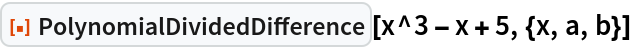Out=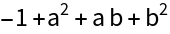Numerically evaluate the divided difference of a polynomial:

 In:=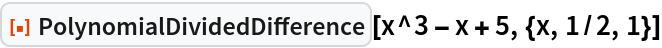Out=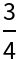### Scope (2)

Divided difference of a polynomial with symbolic coefficients:

 In:=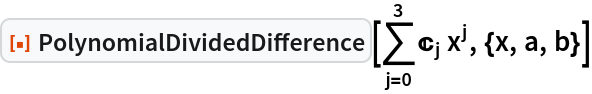Out=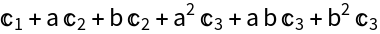Divided difference of a polynomial with numerical coefficients:

 In:=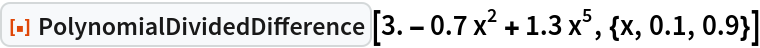Out=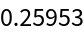### Applications (3)

A high-degree polynomial:

 In:=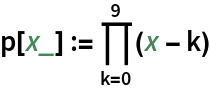Directly evaluating the divided difference through its definition gives a result that is not very accurate:

 In:=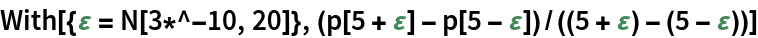Out=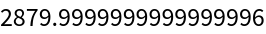PolynomialDividedDifference gives a more accurate result:

 In:=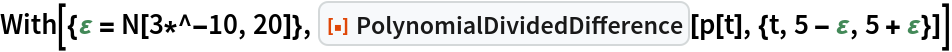Out=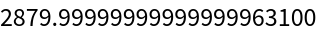Use PolynomialDividedDifference to evaluate a definite integral:

 In:=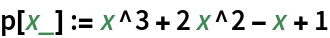In:=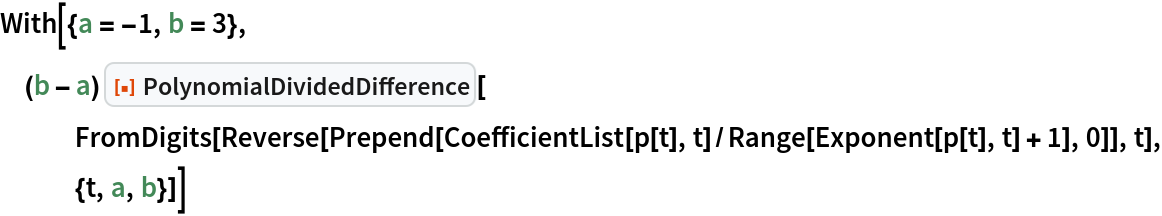Out=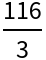Compare with the result using Integrate:

 In:=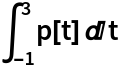Out=Evaluate the q-derivative of a polynomial using PolynomialDividedDifference:

 In:=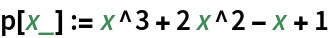In:=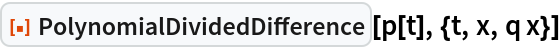Out=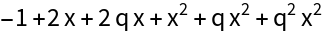In the limit q1, the q-derivative reduces to the derivative:

 In:=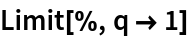Out=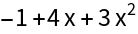In:=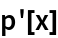Out=### Properties and Relations (1)

When b=a, the divided difference of a polynomial p(x) at a and b is equal to the derivative of p(x), evaluated at x=a:

 In:=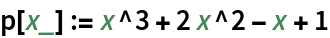In:=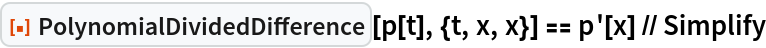Out=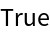## Version History

• 1.0.0 – 31 December 2020